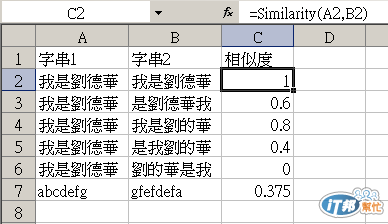#DAY 5
2

## Excel VBA 的眉眉角角Day5:關於function與函數，以相似度程式作為討論

Excel有趣的地方，是它提供了許多內建的函數，可以在每個不同的儲存格內撰寫不同的公式，帶入各式各樣的函數使用。但是函數在怎麼豐富，總有我們缺少的函數，這時該怎麼辦呢？自己寫一個吧！VBA內撰寫function即可由儲存格內帶入自訂函數使用，我們以下撰寫一個「相似度」程式作為討論，以下共分成3個部份，一個主程式「Similarity」兩個子程式「min」與「ld」：

``````Public Function Similarity(str1 As String, str2 As String)
'比較兩字串相似度

Dim ldint As Integer
ldint = ld(str1, str2)
Dim strlen As Integer
If (Len(str1) >= Len(str2)) Then
strlen = Len(str1)
Else
strlen = Len(str2)
End If
Similarity = 1 - ldint / strlen
End Function

Private Function min(one As Integer, two As Integer, three As Integer)
min = one
If (two < min) Then
min = two
End If
If (three < min) Then
min = three
End If
End Function

Private Function ld(str1 As String, str2 As String)
Dim n, m, i, j As Integer
Dim ch1, ch2 As String
n = Len(str1)
m = Len(str2)
Dim temp As Integer
If (n = 0) Then
ld = m
End If
If (m = 0) Then
ld = n
End If
Dim d As Variant
ReDim d(n + 1, m + 1) As Variant
For i = 0 To n
d(i, 0) = i
Next i
For j = 0 To m
d(0, j) = j
Next j
For i = 1 To n
ch1 = Mid(str1, i, 1)
For j = 1 To m
ch2 = Mid(str2, j, 1)
If (ch1 = ch2) Then
temp = 0
Else
temp = 1
End If
d(i, j) = min(d(i - 1, j) + 1, d(i, j - 1) + 1, d(i - 1, j - 1) + temp)
Next j
Next i
ld = d(n, m)
End Function
``````

Public Function Similarity(str1 As String, str2 As String)這行，Public Function的意思，就是讓所有的模組都可以使用，Similarity是該function的名稱，可帶入str1與str2兩個字串，最終取得兩個字串的相似度數值。

min，帶入3個整數，取得最小的整數值。

Ld，其實這才是主要運算的程式，用來計算兩個字串的離異程度，帶出的數值，最後帶回主程式後以一減掉後除以字串長度，即可得知相似度。

``````Sub Similarity_test()
Debug.Print Similarity("我是劉德華", "我是劉德華")
Debug.Print Similarity("我是劉德華", "是劉德華我")
Debug.Print Similarity("我是劉德華", "我是劉的華")
Debug.Print Similarity("我是劉德華", "是我劉的華")
Debug.Print Similarity("我是劉德華", "劉的華是我")
Debug.Print Similarity("abcdefg", "gfefdefa")
End Sub
``````

1

0.6

0.8

0.4

0

0.375Levenshtein distance：http://en.wikipedia.org/wiki/Levenshtein\_distance

Excel VBA 的眉眉角角30